# KSEEB Solutions for Class 8 Maths Chapter 11 Congruency of Triangles Ex 11.3

In this chapter, we provide KSEEB SSLC Class 8 Maths Chapter 11 Congruency of Triangles Ex 11.3 for English medium students, Which will very helpful for every student in their exams. Students can download the latest KSEEB SSLC Class 8 Maths Chapter 11 Congruency of Triangles Ex 11.3 pdf, free KSEEB SSLC Class 8 Maths Chapter 11 Congruency of Triangles Ex 11.3 pdf download. Now you will get step by step solution to each question.

### Karnataka State Syllabus Class 8 Maths Chapter 11 Congruency of Triangles Ex 11.3

Question 1.
In a Δ ABC, AB = AC andlA.= 50° find ∠B and ∠C.
∠A+ ∠B + ∠C = 180°
(Sum of the angles of a triangle is 180° )
50 +∠[B + ∠B = 180°
∠B = ∠C Base angles of an isosceles triangle
50 + 2∠B= 180°
2∠B = 180 – 50
2∠B = 130°
∠B = 130∘2
∠B = 65°
∠B = ∠C = 65°

Question 2.
In AABC,AB = BCand|B = 64°find|£,
AB = BC [data]
∴ ∠C = ∠A [Theorem 1]
∠A + ∠B + ∠C = 180°
(Sum of the angles of a triangle is 180°)
∠C + 64 + ∠C = 180° [∠A = ∠C]
64 + 2∠C = 180°
2∠C = 180 – 64
2∠C = 116
∠C = 1162 = 58°

Question 3.
In each of the following figure find the value of x :
i.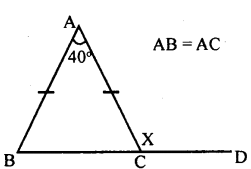In ∆ABC, AB = AC
∴ ∠ABC = ∠ACB
∴ ∠BAC + ∠ABC + ∠ACB = 180°
(Sum of the angles of a triangle is 180°)
40 + ∠ABC + ∠ABC = 180°
(∠ABC =∠ACB)
40 + 2∠ABC = 180°
2∠ABC = 180 – 40
2∠ABC = 140°
∠ABC = 70°
∠ACB = ∠ ABC = 70°
∠ACB + ∠ACD = 180°
70 + x = 180°
x = 180 – 70
x = 110°

ii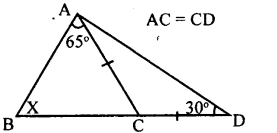AC = CD
∠ACD + ∠CAD + ∠CDA = 180°
(Sum of the angles of a triangle is 180°)
∠ACD + 30 + 30=180°
∠ACD + 60=180°
∠ACD = 180-60
∠ACD = 120°
∠ACD = ∠BAC + ∠ABC
120 = 65° + x
120 – 65 = x
55 = x
x=55°

iiii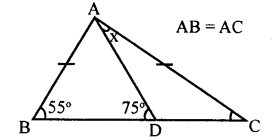AB = AC
∠ABC = ∠ACB = 55° [Theorem l]
Exterior ∠APB = ∠DAC +∠ACD
75 = x + 55
75 – 55 = x
20 = x
x = 20°

iv.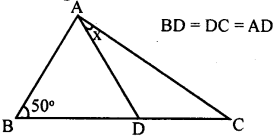BD = DC = AD & ∠ABD = 50 °
50 + 50 +∠ADB = 180°
∠ADB = 180 – 100 = 80°
∠APB = 80°
∴∠DAC = ∠DCA = x°
∴ x + x +∠ADC = 180°
2x + 100 = 180°
2x = 180-100=80°
x=40°

Question 4.
Suppose ABC is an equilateral triangle. Its base BC is produced to D such that BC = CD.
Calculate: l.∠ACD
∠ABC = ∠ACB = ∠BAC = 60°
(ABC is an equilateral triangle)
∠ACB +∠ACD = 180° (Linearpoint)
60 + ∠ACD = 180°
∠ACD = 180 – 60
∠ACD = 120°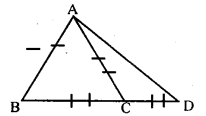In ∆ACD,AC=CD
∠ CAD = ∠CPA (Theorem l)
∠ACB +∠ACD = 180° [linear pair]
60° +∠ACD = 180°
∠ACD = 180° – 60° = 120°
∠ACA +∠CAD + ∠CDA = 180°
2∠CDA = 180 – 120°
2∠CDA = 60°
∠CDA = 602 = 30°
∠CDA = 30

Question 5.
Show that the perpendicular drawn from the vertices of the base of an isosceles triangle to the opposite sides are equal. ,
Data : In ∆ABC, AB = AC,
BD ⊥AC & CE⊥ AB
To prove : BD = CE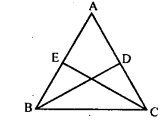Proof:
In ∆ABC, AB = AC [data]
∠ABC = ∠ACB [Theorem l]
In ∆EBC and ∆DCB
∠EBC =∠DCB( Base angles )
∠BEC = ∠CDB [= 90° ]
BC = BC (Common side)
∆EBC = ∆DCB [ASA postulate]
BD = CE [Corresponding sides]

Question 6.
Prove that an ∆ABC is an isosceles triangle if the altitude AD from A on BC bisects BC.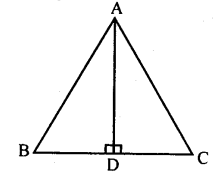BD = DC [AD bisects BC]
∴AB = AC [Correspondingsides]
∴∆ ABC is an isosceles triangle

Question 7.
Suppose a triangle is equilateral, prove that it is equiangular.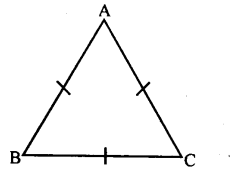To prove: ∠A = ∠B = ∠C
Proof: In ∆ABC, AB = BC
∠C = ∠B [Theorem l]….(i)
BC = AC
∠A =∠B [Theorem l]…(ii)
From (i) and (ii)
∠A =∠B = ∠C
∆ABC is equiangular

All Chapter KSEEB Solutions For Class 8 maths

—————————————————————————–

All Subject KSEEB Solutions For Class 8

*************************************************

I think you got complete solutions for this chapter. If You have any queries regarding this chapter, please comment on the below section our subject teacher will answer you. We tried our best to give complete solutions so you got good marks in your exam.

If these solutions have helped you, you can also share kseebsolutionsfor.com to your friends.

Best of Luck!!